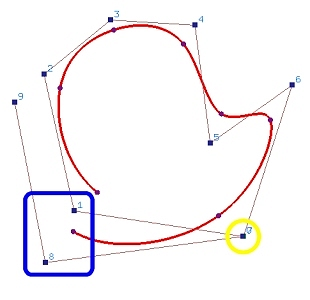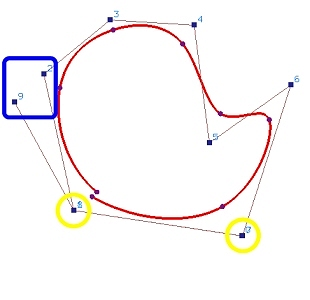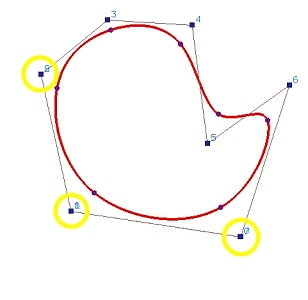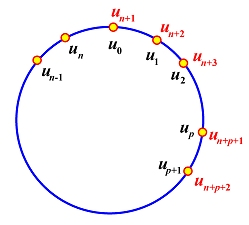# B-spline Curves: Closed CurvesThere are many ways to generate closed curves. The simple ones are either wrapping control points or wrapping knot vectors.### Wrapping Control Points

Suppose we want to construct a closed B-spline curve C(u) of degree p defined by n+1 control points P0, P1, ..., Pn. The number of knots is m+1, where m = n + p + 1. Here is the construction procedure:
1. Design an uniform knot sequence of m+1 knots: u0 = 0, u1 = 1/m, u1 = 2/m, ..., um = 1. Note that the domain of the curve is [up, un-p]. See the discussion in open curves for the details.
2. Wrap the first p and last p control points. More precisely, let P0 = Pn-p+1, P1 = Pn-p+2, ..., Pp-2 = Pn-1 and Pp-1 = Pn. This is shown in the figure below.The constructed curve is Cp-1 continuous at the joining point C(up) = C(un-p).

The following is an example. Figure (a) shows an open B-spline curve of degree 3 defined by 10 (n = 9) control points and a uniform knot vector. In the figure, control point pairs 0 and 7, 1 and 8, and 2 and 9 are placed close to each other to illustrate the construction. Figure (b) shows the result of making control points 0 and 7 identical. The shape of the curve does not change very much. Then, control points 1 and 8 are made identical as shown in Figure (c). It is clear that the gap between the first and last points of the curve is closer. Finally, the curve becomes a closed on when control points 2 and 9 are made identical as shown in Figure (d).(a) (b)(c) (d)### Wrapping Knots

Another way of constructing closed B-spline curves is by wrapping knots. Suppose we want to construct a closed B-spline curve C(u) of degree p defined by n+1 control points P0, P1, ..., Pn. The following is the construction procedure:
1. Add a new control point Pn+1 = P0. Therefore, the number of control points is n+2.
2. Find an appropriate knot sequence of n+1 knots u0, u1, ..., un. These knots are not necessarily uniform, an advantage over the method discussed above.
3. Add p+2 knots and wrap around the first p+2 knots: un+1 = u0, un+2 = u1, ..., un+p = up-1, un+p+1 = up, un+p+2 = up+1 as shown in the following diagram. In this way, we have n+p+2 = (n+1) + p + 1 knots.4. The open B-spline curve C(u) of degree p defined on the above constructed n+1 control points and n+p+2 knots is a closed curve with Cp-1 continuity at the joining point C(u0) = C(un+1). Note that the domain of this closed curve is [u0, un+1].